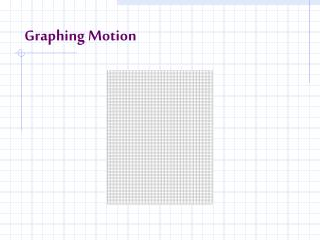DownloadDownload PresentationGraphing Motion

# Graphing Motion

Télécharger la présentation## Graphing Motion

- - - - - - - - - - - - - - - - - - - - - - - - - - - E N D - - - - - - - - - - - - - - - - - - - - - - - - - - -
##### Presentation Transcript

1. Graphing Motion

2. Graphs • Graphs can show how objects change position or speed. (How far away was the turtle after 60 seconds?)

3. Position-Time Graphs • Graphs often show how something changes with time. • This graph shows how temperature changes with time in Santa Barbara, California.

4. Making a Position-Time Graph • This table shows how far a turtle has moved after an amount of time.

5. Making a Position-Time Graph (cont.) • Plotting the time on the x-axis and plotting the distance the turtle has moved on the y-axis creates the graph. • You can draw a line through the points and use it to estimate the turtle’s position at a given time. (Estimate the position of the turtle at 90 seconds)

6. Units on Position-Time Graphs • Each number has units associated with it. • Position has units of length like cm, m, or km. • Seconds, minutes, and days are units of time.

7. Slope of a Position-Time Graph • The steepness of a line on a graph is called the slope. • The steeper the slope, the faster the object is traveling. (Which line represents a faster moving turtle?) • On a position-time graph, a steeper line means a greater average speed.

8. Calculating Slope from a Position-Time Graph • To find the slope of a line, the origin and another point are used to calculate the rise and the run.

9. rise run slope = Calculating Slope from a Position-Time Graph(cont.) • Rise is the change in vertical direction (up and down). • Run is the change in horizontal direction (left to right).

10. Slope and Average Speed • Average speed is the total distance divided by the total time taken to travel that distance. • Rise is equal to the distance traveled. • Run is equal to the time taken to travel that distance. • Average speed is equal to the slope of the line on a position-time graph.

11. Position-Time Graphs for Changing Speed • Only objects with a constant speed will have position-time graphs with a straight line.

12. Position-Time Graphs for Changing Speed(cont.) • To find the average speed of the entire trip, use the starting and ending points.

13. Position-Time Graphs for Changing Speed(cont.) • Then calculate the slope of the line that would connect those points. (Calculate the slope using (0,0) and (10,10))

14. Speed-Time Graphs • Graphing instantaneous speed of an object shows how the speed of an object changes with time. • Constant speed on a speed-time graph is a horizontal line because the speed does not change.

15. Speed-Time Graphs (cont.) • If an object speeds up, the plotted line slants up towards the right.

16. Speed-Time Graphs (cont.) • If an object slows down, the plotted line slants down towards the right.

17. Speed-Time Graphs (cont.)

18. Lesson 1-3: Review The horizontal change on a graph is also called the ____. A rise B elevation C run D distance

19. Lesson 1-3: Review The steepness of a line on a graph is called the ____. A slope B hill C rise D run

20. Lesson 1-3: Review If the rise is equal to 75 m and the run is equal to 2.5 m, then what is the slope? A 187.5 m B 12 m C 30 m D 0.03 cm